# Integration (scipy.integrate)¶

The scipy.integrate sub-package provides several integration techniques including an ordinary differential equation integrator. An overview of the module is provided by the help command:

>>> help(integrate)
Methods for Integrating Functions given function object.

quad          -- General purpose integration.
dblquad       -- General purpose double integration.
tplquad       -- General purpose triple integration.
fixed_quad    -- Integrate func(x) using Gaussian quadrature of order n.
quadrature    -- Integrate with given tolerance using Gaussian quadrature.
romberg       -- Integrate func using Romberg integration.

Methods for Integrating Functions given fixed samples.

trapz         -- Use trapezoidal rule to compute integral from samples.
cumtrapz      -- Use trapezoidal rule to cumulatively compute integral.
simps         -- Use Simpson's rule to compute integral from samples.
romb          -- Use Romberg Integration to compute integral from
(2**k + 1) evenly-spaced samples.

See the special module's orthogonal polynomials (special) for Gaussian
quadrature roots and weights for other weighting factors and regions.

Interface to numerical integrators of ODE systems.

odeint        -- General integration of ordinary differential equations.
ode           -- Integrate ODE using VODE and ZVODE routines.


## General integration (quad)¶

The function quad is provided to integrate a function of one variable between two points. The points can be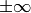(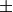inf) to indicate infinite limits. For example, suppose you wish to integrate a bessel function jv(2.5,x) along the interval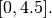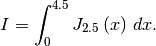This could be computed using quad:

>>> result = integrate.quad(lambda x: special.jv(2.5,x), 0, 4.5)
>>> print result
(1.1178179380783249, 7.8663172481899801e-09)

>>> I = sqrt(2/pi)*(18.0/27*sqrt(2)*cos(4.5)-4.0/27*sqrt(2)*sin(4.5)+
sqrt(2*pi)*special.fresnel(3/sqrt(pi)))
>>> print I
1.117817938088701

>>> print abs(result-I)
1.03761443881e-11


The first argument to quad is a “callable” Python object (i.e a function, method, or class instance). Notice the use of a lambda- function in this case as the argument. The next two arguments are the limits of integration. The return value is a tuple, with the first element holding the estimated value of the integral and the second element holding an upper bound on the error. Notice, that in this case, the true value of this integral is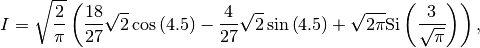where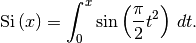is the Fresnel sine integral. Note that the numerically-computed integral is within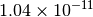of the exact result — well below the reported error bound.

Infinite inputs are also allowed in quad by usinginf as one of the arguments. For example, suppose that a numerical value for the exponential integral: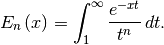is desired (and the fact that this integral can be computed as special.expn(n,x) is forgotten). The functionality of the function special.expn can be replicated by defining a new function vec_expint based on the routine quad:

>>> from scipy.integrate import quad
>>> def integrand(t,n,x):
...     return exp(-x*t) / t**n

>>> def expint(n,x):
...     return quad(integrand, 1, Inf, args=(n, x))

>>> vec_expint = vectorize(expint)

>>> vec_expint(3,arange(1.0,4.0,0.5))
array([ 0.1097,  0.0567,  0.0301,  0.0163,  0.0089,  0.0049])
>>> special.expn(3,arange(1.0,4.0,0.5))
array([ 0.1097,  0.0567,  0.0301,  0.0163,  0.0089,  0.0049])


The function which is integrated can even use the quad argument (though the error bound may underestimate the error due to possible numerical error in the integrand from the use of quad ). The integral in this case is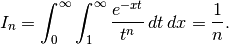>>> result = quad(lambda x: expint(3, x), 0, inf)
>>> print result
(0.33333333324560266, 2.8548934485373678e-09)

>>> I3 = 1.0/3.0
>>> print I3
0.333333333333

>>> print I3 - result
8.77306560731e-11


This last example shows that multiple integration can be handled using repeated calls to quad. The mechanics of this for double and triple integration have been wrapped up into the functions dblquad and tplquad. The function, dblquad performs double integration. Use the help function to be sure that the arguments are defined in the correct order. In addition, the limits on all inner integrals are actually functions which can be constant functions. An example of using double integration to compute several values of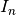is shown below:

>>> from scipy.integrate import quad, dblquad
>>> def I(n):
...     return dblquad(lambda t, x: exp(-x*t)/t**n, 0, Inf, lambda x: 1, lambda x: Inf)

>>> print I(4)
(0.25000000000435768, 1.0518245707751597e-09)
>>> print I(3)
(0.33333333325010883, 2.8604069919261191e-09)
>>> print I(2)
(0.49999999999857514, 1.8855523253868967e-09)


## Gaussian quadrature (integrate.gauss_quadtol)¶

A few functions are also provided in order to perform simple Gaussian quadrature over a fixed interval. The first is fixed_quad which performs fixed-order Gaussian quadrature. The second function is quadrature which performs Gaussian quadrature of multiple orders until the difference in the integral estimate is beneath some tolerance supplied by the user. These functions both use the module special.orthogonal which can calculate the roots and quadrature weights of a large variety of orthogonal polynomials (the polynomials themselves are available as special functions returning instances of the polynomial class — e.g. special.legendre).

## Integrating using samples¶

There are three functions for computing integrals given only samples: trapz , simps, and romb . The first two functions use Newton-Coates formulas of order 1 and 2 respectively to perform integration. These two functions can handle, non-equally-spaced samples. The trapezoidal rule approximates the function as a straight line between adjacent points, while Simpson’s rule approximates the function between three adjacent points as a parabola.

If the samples are equally-spaced and the number of samples available is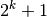for some integer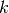, then Romberg integration can be used to obtain high-precision estimates of the integral using the available samples. Romberg integration uses the trapezoid rule at step-sizes related by a power of two and then performs Richardson extrapolation on these estimates to approximate the integral with a higher-degree of accuracy. (A different interface to Romberg integration useful when the function can be provided is also available as romberg).

## Ordinary differential equations (odeint)¶

Integrating a set of ordinary differential equations (ODEs) given initial conditions is another useful example. The function odeint is available in SciPy for integrating a first-order vector differential equation: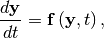given initial conditions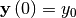, where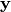is a length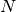vector and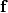is a mapping from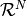to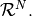A higher-order ordinary differential equation can always be reduced to a differential equation of this type by introducing intermediate derivatives into thevector.

For example suppose it is desired to find the solution to the following second-order differential equation: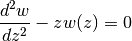with initial conditions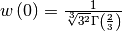and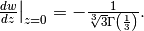It is known that the solution to this differential equation with these boundary conditions is the Airy function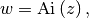which gives a means to check the integrator using special.airy.

First, convert this ODE into standard form by setting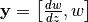and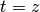. Thus, the differential equation becomes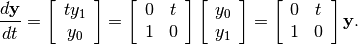In other words,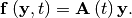As an interesting reminder, if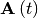commutes with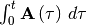under matrix multiplication, then this linear differential equation has an exact solution using the matrix exponential: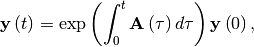However, in this case,and its integral do not commute.

There are many optional inputs and outputs available when using odeint which can help tune the solver. These additional inputs and outputs are not needed much of the time, however, and the three required input arguments and the output solution suffice. The required inputs are the function defining the derivative, fprime, the initial conditions vector, y0, and the time points to obtain a solution, t, (with the initial value point as the first element of this sequence). The output to odeint is a matrix where each row contains the solution vector at each requested time point (thus, the initial conditions are given in the first output row).

The following example illustrates the use of odeint including the usage of the Dfun option which allows the user to specify a gradient (with respect to) of the function,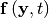.

>>> from scipy.integrate import odeint
>>> from scipy.special import gamma, airy
>>> y1_0 = 1.0/3**(2.0/3.0)/gamma(2.0/3.0)
>>> y0_0 = -1.0/3**(1.0/3.0)/gamma(1.0/3.0)
>>> y0 = [y0_0, y1_0]
>>> def func(y, t):
...     return [t*y,y]

>>> def gradient(y,t):
...     return [[0,t],[1,0]]

>>> x = arange(0,4.0, 0.01)
>>> t = x
>>> ychk = airy(x)
>>> y = odeint(func, y0, t)
>>> y2 = odeint(func, y0, t, Dfun=gradient)

>>> print ychk[:36:6]
[ 0.355028  0.339511  0.324068  0.308763  0.293658  0.278806]

>>> print y[:36:6,1]
[ 0.355028  0.339511  0.324067  0.308763  0.293658  0.278806]

>>> print y2[:36:6,1]
[ 0.355028  0.339511  0.324067  0.308763  0.293658  0.278806]


### Table Of Contents

#### Previous topic

Special functions (scipy.special)

#### Next topic

Optimization (scipy.optimize)